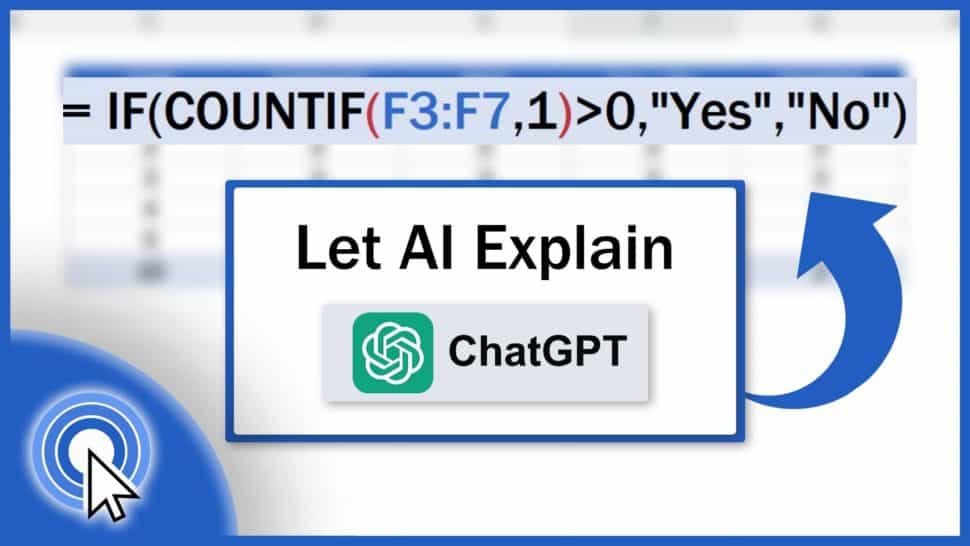# No Stress About Excel Formulas – Let AI Explain (Inside Excel)This tutorial shows how we can use AI, specifically ChatGPT, to help us understand what exactly the Excel formula we just used does. This comes quite handy as we can ask for an explanation right inside Excel. Isn’t that great?

Let’s have a look at how it works on a couple of examples now.

Contents

In the last video we covered how to create Excel formulas – super easy with AI. We had a look at how we can generate an Excel formula in a quick and easy way, based on a simple description of what you need to do in a data table. If you haven’t had time to watch it yet, make sure to do so to get a complete picture of how AI can make work in Excel easier for you. The link to the tutorial is in the list below.

ChatGPT for Excel: How to Create Excel Formulas – Super Easy with AI

## How to Ask AI For An Explanation Of Any Formula

Now, we can move on and have a look at how we can find out what exactly an Excel formula does, thanks to AI. And again, as previously, we’re going to use this amazing ‘AI-Aided Formula Editor’ tool, which is paid, but you can add it to Excel and try it out for free right after you’ve finished watching this tutorial. The step-by-step procedure of how to do that is provided in the last tutorial.

Here we’re going to go through another interesting feature of this AI tool.

So, if we’d like to know what each formula in our spreadsheet does, we’ll use this ‘Cell Formula’ part here.

We’ll leave it on and we’ll hide the formula generator used previously by simply clicking on it.

We also need to click on ‘Console’ here, because we’ll need AI to provide us with the formula explanation in this grey field.

## Analyzing Sum Formula in Excel

So, let’s start analysing various formulas we’ve got here and we can start with something really simple.

We can click on the cell C8 and the formula from this cell gets copied immediately and shows in the editor.

Now we just press this button with a speech bubble and AI provides us with an explanation right away:

‘This Excel formula calculates the sum of the values in the cell range C3 to C7, which includes cells C3, C4, C5, C6, and C7.’

Great! Here we’ve got a clear and specific explanation.

We can use this crossed circle sign to remove the explanation and move on.

## Analyzing Average Formula in Excel

Now we click on D8 where there’s another formula, we use the speech bubble button again, and here we go with another explanation.

‘This Excel formula calculates the average (mean) value of the range of cells from D3 to D7 in the spreadsheet.’

If needed, we can click on the button again and we’ll get another, more detailed explanation, which might cast more light on how things work.

After the second attempt, the explanation comes to this…

Then this…

One more click and we’ve got the most comprehensive answer which is:

‘This Excel formula calculates the average value of the range of cells from D3 to D7, i.e., it adds the values in cells D3, D4, D5, D6, and D7, and then divides the sum by the number of cells (5 in this case) to find the average.’

Perfect! Everything clear.

## Excel Formula Checks If There Is Any Value Equal to 1

Now we can move on to something more complicated. Let’s click on the cell F8 and we can see that the formula there looks a bit more complex.

‘This Excel formula checks if there is any value equal to 1 in the range F3 to F7. If there is at least one value equal to 1 in that range, the formula returns “Yes”, otherwise, it returns “No”.’

Let’s check that out. If we change the value 1 to let’s say number 8 and there will be no value equal to 1 in column F, the formula returns ‘No’.

So, even this explanation is correct and the formula works as described.

You can use the same way to ask AI for an explanation of any formula you need to analyse at that moment.

However, we must remind you to be aware that one cannot rely on AI completely. It’s important to use common sense and bear in mind that AI is still learning, still advancing.

But even now it can be a great help while using Excel.

So, are you ready to give ChatGPT inside Excel a try? Share your ideas with us in the comments section below. We’ll be happy to hear from you!

And, as mentioned before, make sure to see the first part of this series of tutorials about ChatGPT How to Create Excel Formulas – Super Easy With AI, and you’ll see how you can add this amazing tool to Excel in a simple way. The link to the video is in the list below.

Don’t miss out a great opportunity to learn:

If you found this tutorial helpful, give us a like and watch other tutorials by EasyClick Academy. Learn how to use Excel in a quick and easy way!

Is this your first time on EasyClick? We’ll be more than happy to welcome you in our online community. Hit that Subscribe button and join the EasyClickers!

Thanks for watching and I’ll see you in the next tutorial!